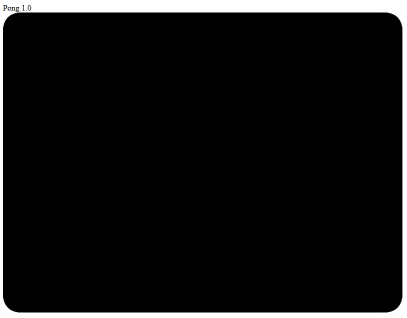# JavaScript: Intro to Web Game Development – Part 2: canvasContext with rounded corners

This is Part 2 of the series where I walk through the steps to create a Pong game in the web browser using JavaScript. Part 1 is here:

JavaScript: Intro to Web Game Development – Part 1: canvas element

In this step, I will create the black background for the pong game, but instead of a perfect rectangle, I am going to give the playing space rounded corners. Since I want to see a fresh copy of the game whenever I refresh my browser, I am going to define a function that launches whenever the window loads using:

`window.onload = function() {}`

Inside this function, I am going to do the following:

• Refer to the canvas object defined in Step 1.
• Create a canvasContext to draw on
• Define canvas_width and canvas_height variables
• Define and call a new function called roundRect which will give the canvasContext rounded corners

Step 1: Refer to the canvas object defined in the HTML

`canvas = document.getElementById("gameCanvas")`

Step 2: Create a canvasContext

Set the canvasContext using `getContext()`, with a value of “2d”:

`canvasContext = canvas.getContext("2d");`

The canvasContext is the drawing context of the canvas. For more info about  `canvas.getContext`, see:
https://developer.mozilla.org/en-US/docs/Web/API/HTMLCanvasElement/getContext

“2d” is the `contextType`. Setting this creates a `CanvasRenderingContext2D` object representing a 2d rendering context. For more information about CanvasRenderingContext2D, see:
https://developer.mozilla.org/en-US/docs/Web/API/CanvasRenderingContext2D

Step 3: Set height and width variables

Set the canvas_width and canvas_height variables with the canvas width and height:

```var canvas_width = canvas.width;
var canvas_height = canvas.height;```

Step 4: Define and call a new function called roundRect

The function roundRect will inherit from the CanvasRenderingContext2D object, which is why this function is defined using .prototype. For example: `CanvasRenderingContext2D.prototype.roundRect`:

`CanvasRenderingContext2D.prototype.roundRect = function(x, y, width, height, radius, fill, stroke) {`

“prototype” is used for object inheritance:
http://javascriptissexy.com/javascript-prototype-in-plain-detailed-language/

The roundRect function will use a path to define a rectangle canvas shape with rounded corners. This function will take in the following variables when called:

`x, y, width, height, radius, fill, stroke`
• x and y locations for where the roundRect will begin
• the width and height of the rectangle to create
• the radius of the circle that defines the rounded curves
• the color of the fill
• the style of the stroke

This function works on a canvasContext, which is derived from an HTML canvas. For example, we can call the roundRect function to give the gameCanvas rounded corners with a radius of 40 and a fill color of black:

`canvasContext.roundRect(0, 0, canvas_width, canvas_height, 40, "black");`

The roundRect function is necessary because there are no built-in functions for making a canvas with rounded corners. What the roundRect function does is create a path using the HTML5 canvas `beginPath()`, `closePath()`, `moveTo()`, `lineTo()`, and `quadraticCurveTo()` methods:

```beginPath()

// make some connections that define a shape using
// .moveTo()
// .lineTo()

closePath()```

Here is the complete JavaScript code so far (placed in the <script> portion of the HTML document), with comments:

```var canvas;

canvas = document.getElementById("gameCanvas")
canvasContext = canvas.getContext("2d");

var canvas_width = canvas.width;
var canvas_height = canvas.height;

CanvasRenderingContext2D.prototype.roundRect = function(x, y, width, height, radius, fill, stroke) {

// If stroke argument not provided, provide a stroke by default
if (typeof stroke == "undefined" ) {
stroke = true;
}
// If no radius is defined, then give it a default of 5
if (typeof radius === "undefined") {
}

// Start of shape definition
this.beginPath();

// Draw the top border line
this.lineTo(x + width - radius, y);

// Draw the top right curve

// Draw the right border line
this.lineTo(x + width, y + height - radius);

// Draw the bottom right curve
this.quadraticCurveTo(x + width, y + height, x + width - radius, y + height);

// Draw the bottom border line
this.lineTo(x + radius, y + height);

// Draw the bottom left curve

// Draw the left border line

// Draw the final (top left) curve

// End of shape definition
this.closePath();

// Draw the shape with a line
if (stroke) {
this.stroke();
}
// Fill in the shape with color
if (fill) {
this.fill();
}
}

// Now call the function
canvasContext.roundRect(0, 0, canvas_width, canvas_height, 40, "black");

}```

Here is what the canvas looks like so far: